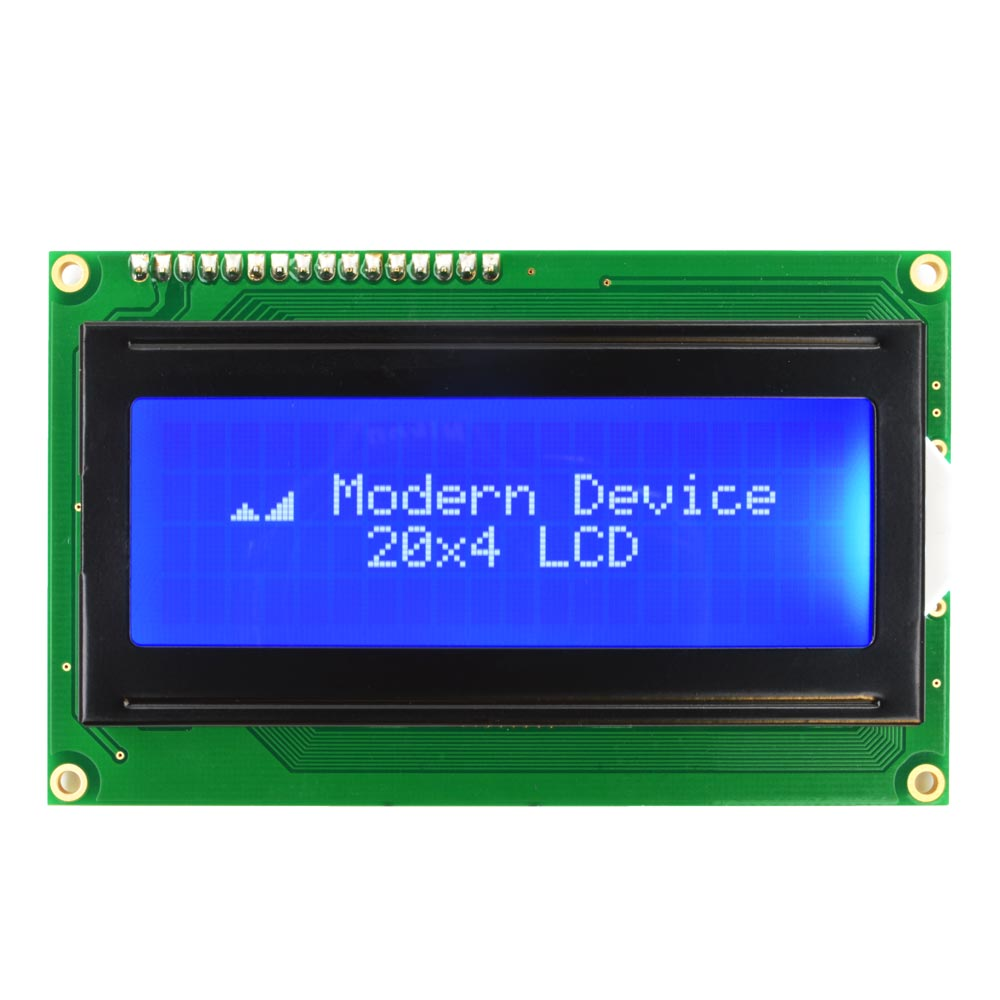Skip to ContentDan k
Chen
Big LCD Clock
Explorations Dan Chen / January 11, 2015

I was in the store and saw the MUJI brand big LCD clock but didn’t want to pay \$40 for it, so I decided to make the clock with the parts that I have around in the LAB.

I’ve got the LCD from http://moderndevice.com/product/lcd117-bundle/with the serial backpack. You can get the LCD and serial backpack from Sparkfun or http://adafruit.com as well.```#include

int sec=0;

int hour=1;
int minute=5;
String ampm="PM";

boolean needclear=true;

#define rxPin 4  // rxPin is immaterial - not used - just make this an unused Arduino pin number
#define txPin A0 // pin 14 is analog pin 0, on a BBB just use a servo cable :), see Reference pinMode
SoftwareSerial mySerial =  SoftwareSerial(rxPin, txPin);

void setup(){

pinMode(txPin, OUTPUT);
mySerial.begin(9600);      // 9600 baud is chip comm speed
int senVal=0;

//hide course
mySerial.print("?c0");
// set LCD brightness
mySerial.print("?B20");

}

void loop(){

sec++;

if (sec==60){
minute++;
sec=0;
needclear=true;
}
if (minute==60){
hour++;
minute=0;
}
if (hour==13){
hour=1;
if (ampm=="PM"){ampm="AM";}
if (ampm=="AM"){ampm="PM";}
}

if (needclear){
//clear the LCD
mySerial.print("?f");

// enter big number mode
mySerial.print("?>3");

if (hour<10){mySerial.print("0");}
mySerial.print(hour);
mySerial.print(":");
if (minute<10){mySerial.print("0");}
mySerial.print(minute);

//ext big number mode
mySerial.print("?<");
mySerial.print(ampm);
needclear=false;
}
delay(1000);

}
```

The LCD also work with cheap and cheerful Trinket from Adafruit. I was going to use the on board RED led as the second hand, however I can’t get it to blink and keep the time at the same time. But the below code works with Trinket. Just connect the signal pin to #3.```#include

int sec=0;

int hour=5;
int minute=50;
String ampm="PM";

boolean needclear=true;

#define rxPin 4  // rxPin is immaterial - not used - just make this an unused Arduino pin number
#define txPin 3 // pin 14 is analog pin 0, on a BBB just use a servo cable :), see Reference pinMode
SoftwareSerial mySerial =  SoftwareSerial(rxPin, txPin);

void setup(){

pinMode(txPin, OUTPUT);
mySerial.begin(9600);      // 9600 baud is chip comm speed
int senVal=0;
//hide course

mySerial.print("?c0");
mySerial.print("?B20");

}

void loop(){

sec++;

if (sec==60){
minute++;
sec=0;
needclear=true;
}
if (minute==60){
hour++;
minute=0;
}
if (hour==13){
hour=1;
if (ampm=="PM"){ampm="AM";}
if (ampm=="AM"){ampm="PM";}
}

if (needclear){
//clear the LCD

mySerial.print("?f");

// enter big number mode
mySerial.print("?>3");

if (hour<10){mySerial.print("0");}
mySerial.print(hour);

mySerial.print(":");

if (minute<10){mySerial.print("0");}
mySerial.print(minute);

//ext big number mode
mySerial.print("?<");
mySerial.print(ampm);
needclear=false;
}

delay(1000);

/*
for (byte i=0; i<256; i++){      mySerial.print("?B");       mySerial.print(i, HEX);     }    */    /*    for (byte i=255; i>0; i--){
mySerial.print("?B");
mySerial.print(i, HEX);
}

*/

}

```